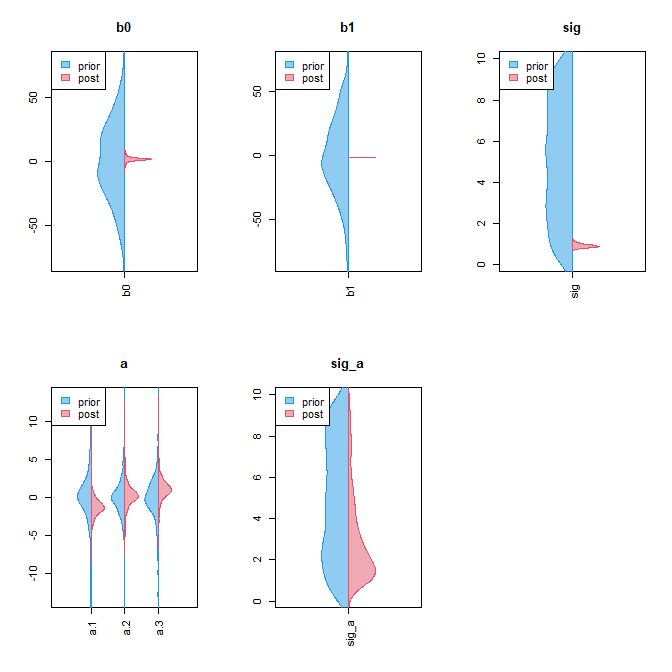# jagshelper-vignette

library(jagshelper)

# jagshelper

The goal of jagshelper is to streamline Bayesian analysis in JAGS using the jagsUI package.
Functions are provided for extracting output in a simpler form, assessing model convergence, and plotting model output. Also included is a function giving a template model in JAGS syntax with the associated jagsUI code.

Some of the jagshelper functionality is illustrated below, with steps approximately corresponding to those of a typical Bayesian analysis using JAGS.

## Model template

When starting a Bayesian analysis in JAGS from scratch, I can never remember the exact structure for doing so, and sometimes need reminding of basic JAGS model syntax.

The skeleton() function prints a JAGS model template to the screen, along with code to simulate a corresponding dataset.

library(jagshelper)
skeleton("EXAMPLE")
#>
#> library(jagsUI)
#>
#> # specify model, which is written to a temporary file
#> EXAMPLE_jags <- tempfile()
#> cat('model {
#>   for(i in 1:n) {
#>     y[i] ~ dnorm(mu[i], tau)
#>     mu[i] <- b0 + b1*x[i] + a[grp[i]]
#>   }
#>
#>   for(j in 1:ngrp) {
#>     a[j] ~ dnorm(0, tau_a)
#>   }
#>
#>   tau <- pow(sig, -2)
#>   sig ~ dunif(0, 10)
#>   b0 ~ dnorm(0, 0.001)
#>   b1 ~ dnorm(0, 0.001)
#>
#>   tau_a <- pow(sig_a, -2)
#>   sig_a ~ dunif(0, 10)
#> }', file=EXAMPLE_jags)
#>
#>
#> # simulate data to go with the example model
#> n <- 60
#> x <- rnorm(n, sd=3)
#> grp <- sample(1:3, n, replace=T)
#> y <- rnorm(n, mean=grp-x)
#>
#> # bundle data to pass into JAGS
#> EXAMPLE_data <- list(x=x,
#>                  y=y,
#>                  n=length(x),
#>                  grp=as.numeric(as.factor(grp)),
#>                  ngrp=length(unique(grp)))
#>
#> # JAGS controls
#> niter <- 10000
#> ncores <- 3
#> # ncores <- min(10, parallel::detectCores()-1)
#>
#> {
#>   tstart <- Sys.time()
#>   print(tstart)
#>   EXAMPLE_jags_out <- jagsUI::jags(model.file=EXAMPLE_jags, data=EXAMPLE_data,
#>                              parameters.to.save=c("b0","b1","sig","a","sig_a"),
#>                              n.chains=ncores, parallel=T, n.iter=niter,
#>                              n.burnin=niter/2, n.thin=niter/2000)
#>   print(Sys.time() - tstart)
#> }

## Output summary & Assessing convergence

### A simple model

Having run a model for the first time, it can be useful to see how many parameter nodes have been saved in total, or how many nodes exist for each named parameter. This can aid in deciding the appropriate strategy for assessing convergence: for example, whether trace plots can be feasibly assessed for all parameter nodes in the model, or just a subset.

In the example below, there are relatively few parameters saved and all trace plots can be examined.

nparam(asdf_jags_out)  # how many parameters in total
#>  8
nbyname(asdf_jags_out)  # how many parameters (or dimensions) per parameter name
#> $b0 #>  1 #> #>$b1
#>  1
#>
#> $sig #>  1 #> #>$a
#>  3
#>
#> $sig_a #>  1 #> #>$deviance
#>  1
tracedens_jags(asdf_jags_out, parmfrow=c(3,3))  # trace plots for all parameters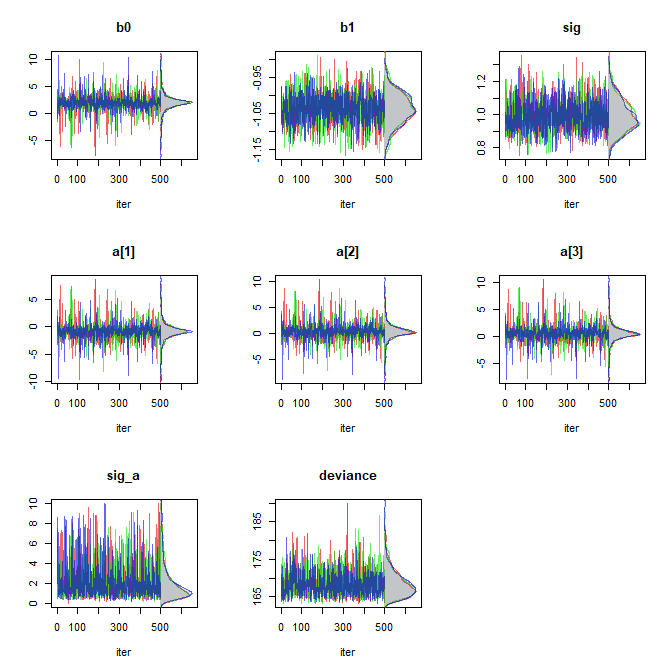check_Rhat(asdf_jags_out)  # proportion of Rhats below a threshold of 1.1
#>       b0       b1      sig        a    sig_a deviance
#>        1        1        1        1        1        1
asdf_jags_out$Rhat # Rhat values #>$b0
#>  0.999954
#>
#> $b1 #>  1.00248 #> #>$sig
#>  1.00394
#>
#> $a #>  1.0002087 0.9995671 1.0000089 #> #>$sig_a
#>  1.000986
#>
#> $deviance #>  1.001197 ### A more complex model In the example below, there are many more parameters saved, and it is perhaps more illustrative to examine the trace plots associated with the least- converged parameter nodes, as measured by Rhat value (Gelman & Rubin 1992). nparam(SS_out) # how many parameters in total #>  334 nbyname(SS_out) # how many parameters (or dimensions) per parameter name #>$trend
#>  41
#>
#> $rate #>  41 #> #>$ypp
#>  41
#>
#> $fit #>  41 #> #>$sig_eps
#>  1
#>
#> $sig_xi #>  1 #> #>$sig_omega
#>  2
#>
#> $cycle #>  41 #> #>$cycle_s
#>  41  2
#>
#> $ar1 #>  41 #> #>$phi
#>  1
#>
ts_postpred(ypp=SS_out, p="ypp", y=SS_data$y)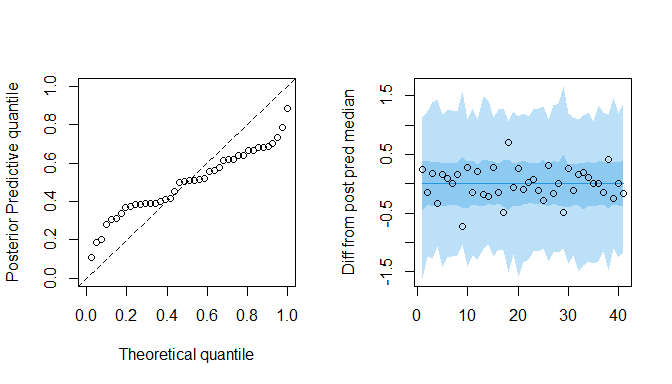par(mfrow=old_parmfrow) # restoring previous graphics state • The function pairstrace_jags() gives methods for plotting two-dimensional trace plots, scatter plots, or contour plots, in which each possible pairing of parameter nodes are plotted with respect to one another. In addition to convergence, this may provide a graphical check for correlation between parameter nodes, or problematic posterior surface shapes. An example is shown below. pairstrace_jags(asdf_jags_out, p=c("a","sig_a"), points=TRUE, parmfrow=c(3,2))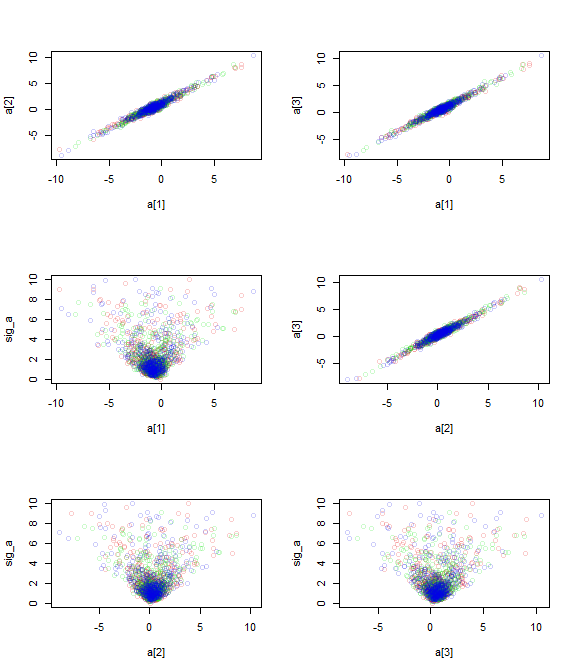• The functions cor_jags() and plotcor_jags() respectively return and plot correlation matrices of all or a subset of parameters, which may be useful in directly assessing correlation between parameters. In the case of multiple nodes per parameter (as in a vector or array of nodes), the full collection of nodes per parameter name is given one axis tick. This is intended to reduce graphical clutter as well as giving greater visual weight to single parameters. An example is given below. plotcor_jags(SS_out, p=c("trend","rate","sig"))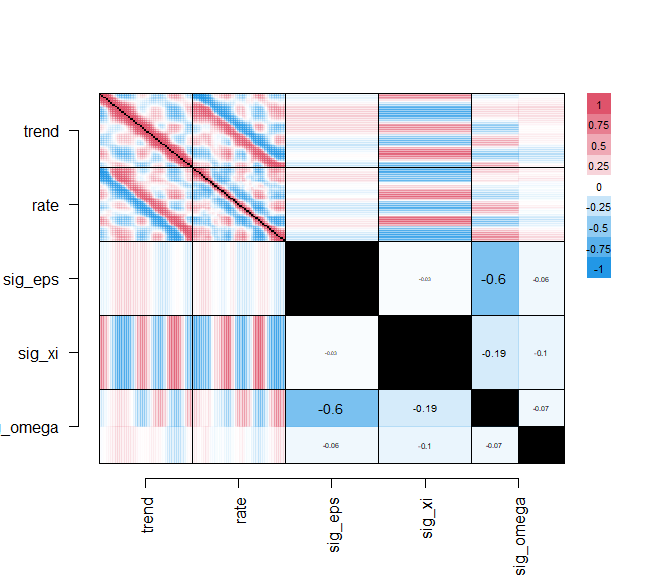## Extracting output as data.frame The jags_df() function extracts all posterior samples from an output object returned by jagsUI::jags() as a data.frame, which may be preferable for some users. out_df <- jags_df(asdf_jags_out) str(out_df) #> 'data.frame': 1500 obs. of 8 variables: #>$ b0      : num  -1.27 -1.54 1.43 1.88 1.35 ...
#>  $b1 : num -0.976 -1.075 -1.031 -1.035 -1.048 ... #>$ sig     : num  0.945 1.213 1.063 1.179 1.12 ...
#>  $a : num 1.7815 3.0941 -0.2099 -0.7276 0.0799 ... #>$ a    : num  3.397 4.155 0.687 0.47 0.682 ...
#>  $a : num 3.747 4.351 1.18 0.349 1.247 ... #>$ sig_a   : num  6.538 5.047 0.82 0.526 0.708 ...
#>  $deviance: num 171 174 165 171 169 ... ### Additional functionality • Functions for trace plots and by-chain kernel density are available with trace_df() and chaindens_df(), respectively, if model output is only saved in data.frame form. Note that both functions contain an additional nline= argument corresponding to the number of MCMC chains that were run. • Functions trace_line() and chaindens_line() are also included for completeness, which take a single vector of MCMC iterations (associated with a single parameter) as input. Note that both functions contain an additional nline= argument corresponding to the number of MCMC chains that were run. ## Plotting a matrix associated with a vector of parameter nodes ### A single matrix The caterpillar() and envelope() functions plot output associated with a vector of parameter nodes. This is often expressed as a two-dimensional matrix or data.frame, with a column for each parameter node and a row for each MCMC iteration. Both caterpillar() and envelope() were originally written to accept such data.frames as inputs, but now also accept a jagsUI output object and parameter name. It is anticipated that caterpillar() could be used for effect sizes associated with a categorical variable, in which plotting order may or may not matter. By contrast, envelope() is intended for a matrix associated with a sequence of parameter nodes, such as in a time series. For a simpler case, plotdens() produces a kernel density plot of a single parameter node, or overlays multiple parameter nodes from a list. Alternately (shown below) it overlays kernel density plots of a vector of parameter nodes. old_parmfrow <- par("mfrow") # storing old graphics state par(mfrow=c(3,1)) caterpillar(asdf_jags_out, "a") envelope(SS_out, "trend", x=SS_data$x)
plotdens(asdf_jags_out, "a")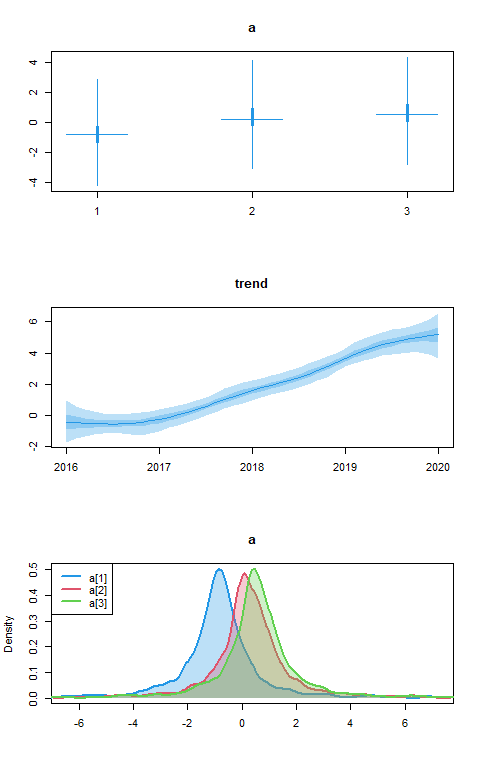par(mfrow=old_parmfrow)  # resetting graphics state

### Multiple matrices or multiple models

It may be appropriate to make by-element comparisons of multiple such matrices, perhaps between multiple candidate models.

Function comparecat() produces interleaved caterpillar plots for a list of jagsUI output objects and an optional list of parameters, plotting parameters common to a set of models adjacent to one another. The example below uses the same output object three times, but will show functionality.

Function comparedens() behaves similarly, but produces left- and right-facing kernel density plots for TWO jagsUI output objects and an optional list of parameters. The example below uses the same output object twice, but will show functionality.

old_parmfrow <- par("mfrow")  # storing old graphics state
par(mfrow=c(2,1))
comparecat(x=list(asdf_jags_out, asdf_jags_out, asdf_jags_out),
p=c("a","b","sig"))
comparedens(x1=asdf_jags_out, x2=asdf_jags_out, p=c("a","b","sig"))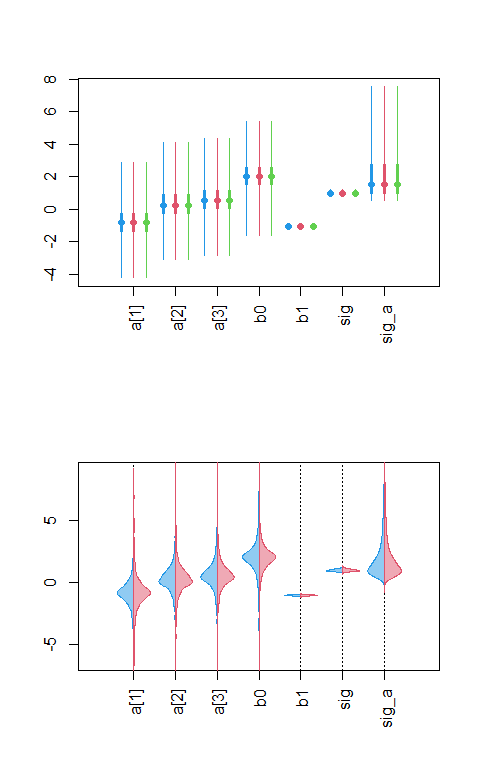par(mfrow=old_parmfrow)  # resetting graphics state

Function overlayenvelope() will automatically overlay multiple envelope() plots, and may be used with a variety of input structures:

• A list() of 2-dimensional posterior data.frames or matrices

• A 3-dimensional array, in which multiple 2-dimensional posterior matrices are joined along the third dimension

• A list() of jagsUI output objects, plus a parameter name

• A single jagsUI output objects, plus a vector of parameter names

par(mfrow=c(2,2))

## usage with list of input data.frames
overlayenvelope(df=list(SS_out$sims.list$cycle_s[,,1],
SS_out$sims.list$cycle_s[,,2]))

## usage with a 3-d input array
overlayenvelope(df=SS_out$sims.list$cycle_s)

## usage with a jagsUI output object and parameter name (2-d parameter)
overlayenvelope(df=SS_out, p="cycle_s")

## usage with a single jagsUI output object and multiple parameters
overlayenvelope(df=SS_out, p=c("trend","rate"))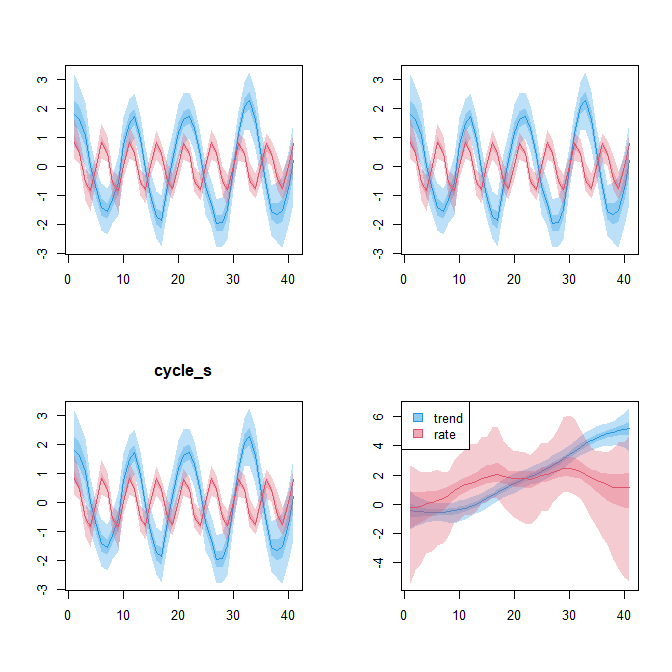### Comparison between priors and posteriors

Function comparepriors() is a wrapper for comparedens(), and plots side-by-side kernel densities of all parameters with names ending in "_prior", along with the respective posterior densities. It should be noted that additional parameters must be included in the JAGS model to provide samples of the prior distributions, as is shown in the example below.

...
sig ~ dunif(0, 10)   # this is the parameter that is used elsewhere in the model
sig_prior ~ dunif(0, 10)  # this is only used to give samples of the prior
...
comparepriors(asdf_prior_jags_out, parmfrow=c(2,3))